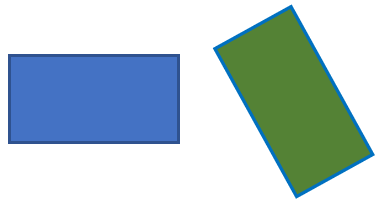# Math Worksheets Land

Math Worksheets For All Ages

# Math Worksheets Land

Math Worksheets For All Ages

# Two-dimensional Congruent Figures Worksheets

When separate geometric figures are congruent, they possess the same shape and size. You can make congruent figures into one another by simply moving them through a rotation, slide, reflection, or another form of translation. For this reason, these figures are said to have congruency. This because an important trait be able to identify and understand when it comes to geometric. Once we identify that this exists, we can better just about any geometric relationships that may be present in the systems that we are examining. If either of these two geometric shapes were to be enlarged or shrunk yet retain the same shape, we could refer to the relationship between them as similar and no longer congruent. That is because their size is now different even though they share the same shape. With these worksheets and lessons, we are learning how to identify if two two-dimensional shapes are actually congruent to one another.

### Aligned Standard: Grade 8 Geometry - 8.G.A.2

• Answer Keys - These are for all the unlocked materials above.

### Homework Sheets

I made all different kinds of shapes on coordinate grids for you to enjoy.

• Homework 1 - These figures are congruent since figure B is producedby translating each vertex of Figure A 4 to the right and 2 up.
• Homework 2 - If it were a paper airplane, I would say that someone pushed it up. The figures are the same size, just moved. So, these figures are congruent since B was produced by translating each vertex of Figure B' 2 to the up and 6 right.
• Homework 3 - If this were a triangular box, it would look as if the box fell on its right side or turned. In this figure, Figure B is a rotation of 90° of Fig A. So, the transformation is a rotation.

### Practice Worksheets

I ask students to explain the transformations of the figures in this part.

• Practice 1 - Describe the sequence of transformations that take place from Figure A to Figure B.
• Practice 2 - A translation is a transformation that slides each point of a figure the same distance in the same direction. It basically slides the figure in on direction or another.
• Practice 3 - These are bit more complex to attack.

### Math Skill Quizzes

Each quiz focuses on a different questioning technique. See how it works for you.

• Quiz 1 - Note the point of origin through out these shapes and placements.
• Quiz 2 - Make sure you identify the shapes and their properties to help you through these.
• Quiz 3 - If we transform the Figure B at the point (-2,-6) at 90° right then what will be the points?

### What are Two-dimensional Congruent Figures?

You must have come across some identical twins, right? If not, let's understand what identical twins are. They are EXACTLY the same! From their eyes to their hair and every other body part, they are totally similar to each other. In math, we refer to this similarity as "Congruency."

Just like identical twins, shapes and figures can be the same too. Let's say you drew a rectangle on one page of 6 cm length 5 cm height. On the next page, you drew another, slightly slant rectangle with the same measurements. These figures are now called congruent to each other. They can even be different colors, but as long as their shape and size are the same, they are congruent.Hence when two figures are congruent, they have the same shape and the same size. Sometimes they may appear rotated or shifted, but all their sides are equal to each other. You will find that most designs are based on the concept of congruent shapes to help maintain a high level of structural integrity. This creates a balance within designs that creates a form of symmetry within a structure. The structural integrity of most physical structure is due to the maintenance of congruence within the design as well. As we get further along with geometry, we will rely on this characteristic between two structures to identify equal sides or angles. This is one of the keys that the foundation of geometry is built upon. In some circumstances we will be told that this relationship exists and in others we will need to prove them.

Unlock all the answers, worksheets, homework, tests and more!
Save Tons of Time! Make My Life Easier Now

## Thanks and Don't Forget To Tell Your Friends!

I would appreciate everyone letting me know if you find any errors. I'm getting a little older these days and my eyes are going. Please contact me, to let me know. I'll fix it ASAP.